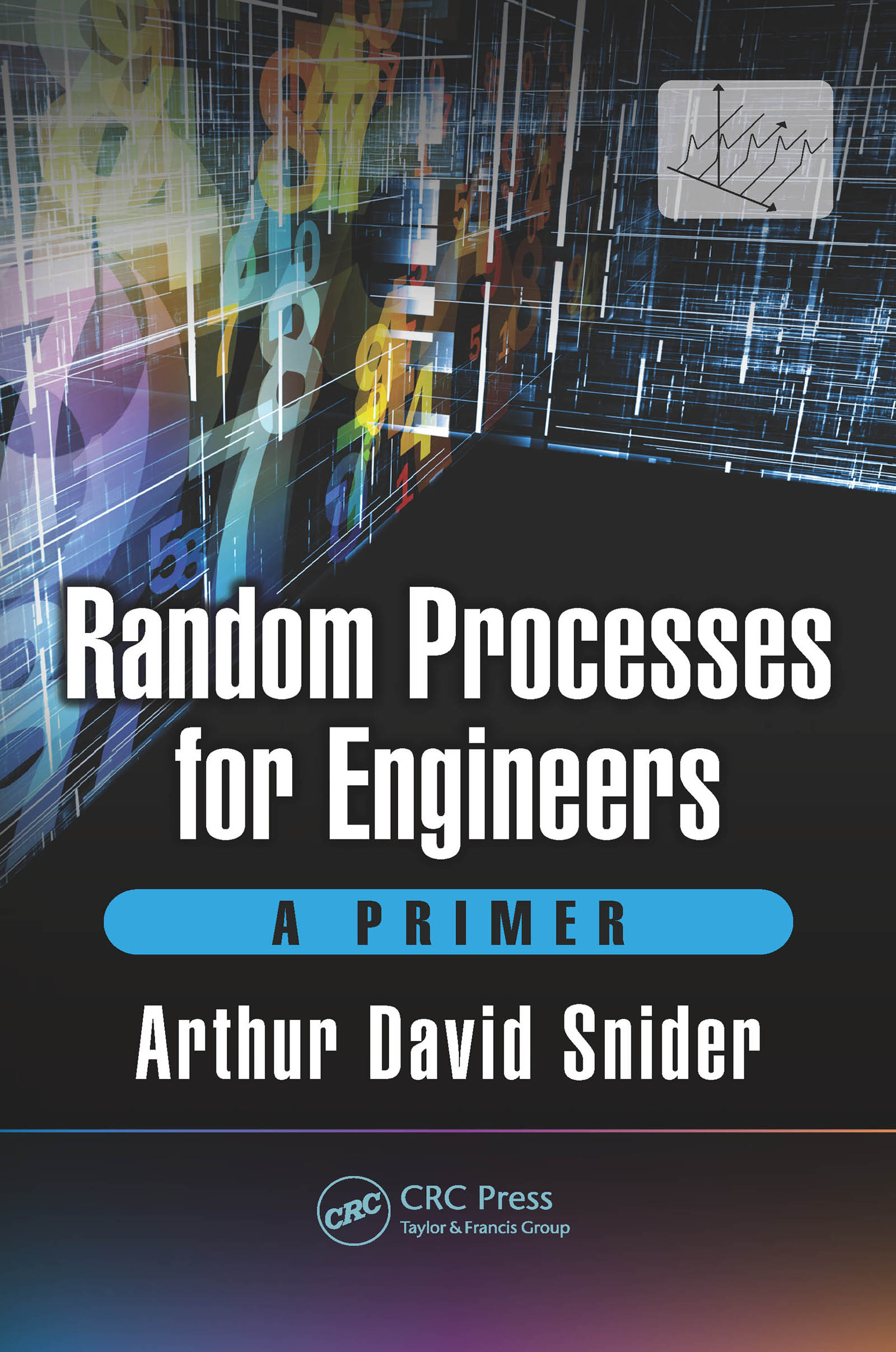Random Processes for Engineers

A Primer, 1st Edition

CRC Press

195 pages | 55 Color Illus. | 28 B/W Illus.

Purchasing Options:\$ = USD
Hardback: 9781498799034
pub: 2017-01-19
SAVE ~\$29.00
\$145.00
\$116.00
x
eBook (VitalSource) : 9781315365480
pub: 2017-01-27
from \$72.50

FREE Standard Shipping!

Description

This book offers an intuitive approach to random processes and educates the reader on how to interpret and predict their behavior. Premised on the idea that new techniques are best introduced by specific, low-dimensional examples, the mathematical exposition is easier to comprehend and more enjoyable, and it motivates the subsequent generalizations. It distinguishes between the science of extracting statistical information from raw data--e.g., a time series about which nothing is known apriori--and that of analyzing specific statistical models, such as Bernoulli trials, Poisson queues, ARMA, and Markov processes. The former motivates the concepts of statistical spectral analysis (such as the Wiener-Khintchine theory), and the latter applies and interprets them in specific physical contexts. The formidable Kalman filter is introduced in a simple scalar context, where its basic strategy is transparent, and gradually extended to the full-blown iterative matrix form.

Reviews

"This is great and timely book! It takes difficult concepts and distills them to the reader in a way that is simple and easy to understand. It connects students with hard to understand theories and concepts though the use of good examples and graphical illustrations."

George Edwards, University of Denver, USA

"This book offers an intuitive approach to random processes and discusses how to interpret and predict their behavior. Based on the idea that new techniques are best introduced by specific, low-dimensional examples, the mathematical exposition is made easier to comprehend and serves to motivate the subsequent generalizations. It distinguishes between the science of extracting statistical information from raw data such as a time series about which nothing is known a priori and that of analyzing specific statistical models, such as Bernoulli trials, Poisson queues, ARMA, and Markov processes. The former motivates the concepts of statistical spectral analysis (such as the Wiener–Khintchine theory), and the latter applies and interprets them in specific physical contexts. The Kalman filter is introduced in a simple scalar context, where its basic strategy is transparent and gradually extended to the full-blown iterative matrix form."

IEEE Control Systems Magazine, December 2017 Issue

Preface

Chapter 1 Probability Basics: A Retrospective

1.1 What Is "Probability"?

1.2 The Additive Law

1.3 Conditional Probability and Independence

Summary: Importat Laws of Probability

1.4 Permutations and Combinations

1.5 Continuous Random Variables

Summary: Important facts About Continuous RandomVariables

1.6 Countability and Measure Theory

1.7 Moments

Summary: Important facts About Expected Values and Moments

1.8 Derived Distributions

Summary: Important Facts About Change of Variable

1.9 The Normal or Gaussian Distribution

Summary: Important Equations Involving the Normal (Gaussian) Distribution

1.10 Multivariate Statistics

1.11 Bivariate probability density functions

Summary: Important Equations for Bivariate Random Variables

1.12 The Bivariate Gaussian Distribution

Summary: Important Equations for the Bivariate Gaussian

1.13 Sums of Random Variables

Summary: Important Equations for Sums of Random Variables

1.14 The Multivariate Gaussian

1.15 The Importance of the Normal Distribution

Exercises for Chapter 1

Chapter 2 Random Processes

2.1 Examples of random processes

2.2 The Mathematical Characterization of Random Processes

Summary: The First and Second Moments of Random Processes

2.3 Prediction: The Statistician's Task

Exercises for Chapter 2

Chapter 3 Analysis of Raw Data

3.1 Stationarity and Ergodicity

3.2 The Limit Concept in Random Processes

3.3 Spectral Methods for Obtaining Autocorrelations

3.4 Interpretation of the Discrete Time Fourier Transform

3.5 The Power Spectral Density

3.6 Interpretation of the Power Spectral Density

3.7 Engineering the Power Spectral Density

3.8 Back to Estimating the Autocorrelation

3.9 The Secret of Bartlett's Method

3.10 Spectral Analysis for Continuous Random Processes

Summary: Spectral Properties of Discrete and Continuous Random Processes

Exercises for Chapter 3

Chapter 4. Models for Random Processes

4.1 Differential Equations Background

4.2 Difference Equations

4.3 ARMA Models

4.4 The Yule-Walker Equations

4.5 Construction of ARMA Models

4.6 Higher-Order ARMA Processes

4.7 The Random Sine Wave

4.8 The Bernoulli and Binomial Processes

Summary: Bernoulli Process

Summary: Binomial Process

4.9 Shot Noise and the Poisson Process

4.10 Random Walks and the Wiener Process

4.11 Markov Processes

Summary: Common Random Process Models

Exercises for Chapter 5

Chapter 5. Least Mean-Square Error Predictors

5.1 The Optimal Constant Predictor

5.2 The Optimal Constant-Multiple Predictor

5.3 Digression: Orthogonality

5.4 Multivariate LMSE Prediction: The Normal Equations

5.5 The Bias

5.6 Best Straight-Line Predictor

5.7 Prediction for a Random Process

5.8 Interpolation, Smoothing, Extrapolation, and Back-Prediction

5.9 The Wiener Filter

Exercises for Chapter 5

Chapter 6 The Kalman Filter

6.1 The Basic Kalman Filter

6.2 Kalman Filter with Transition: Model and Examples

6.3 The Scalar Kalman Filter with Noiseless Transition

6.4 The Scalar Kalman Filter with Noisy Transition

6.5 Iteration of the Scalar Kalman Filter

6.6 Matrix Formulation for the Kalman Filter

Exercises for Chapter 6